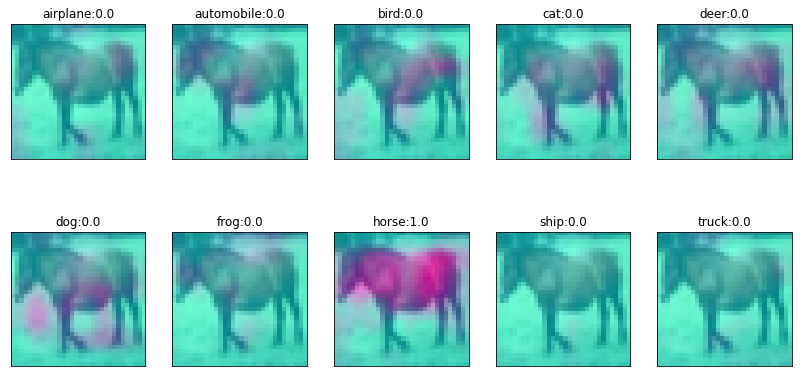# How Deep Neural Networks “See”​ The World

Original article was published on Artificial Intelligence on Medium# How Deep Neural Networks “See”​ The World

Visualization methods are vital to understand how deep networks work. In this article i’ll show you a Simple and intuitive visualization method of how deep networks “see”​ the world.

Let’s describe the methodology behind this Visualization.

Both Average pooling and Max pooling layers operate on filter size. Average Pooling layer works similar to max pooling layer. However, instead of replacing entire areas with the maximum value, it replaces it with the average.

Global Average Pooling layer: Similar to the Average Pooling layer, however it takes the average of all values across the entire feature map (this why it is called global).

Fully Convolutional Networks: With fully connected (Dense) layers, it is impossible to have inputs of different sizes because it will not be compatible with the weight matrices. If all the layers are convolutional, then the number of filter weights is independent of the input image size. However, the the output shape depends on the input size in this case. The only layers that depend on the size of the input are the dense layers.

# #1: Heat-maps

In a classification scenario, if we design the network architecture so that the final convolution consists of filters equal to the number of classes, this will force the final convolutional filters to find out how each part of the image related to each class. Moreover, we can visualize the activations as a heatmap over the input image.

After this final convolution layer, global average pooling is applied across number of convolution features equal to number of classes, which results in a vector of values that we can then use to classify the input image.

# #2: Dataset

Here we used CIFAR-10 dataset consists of 60000 32×32 colour images in 10 classes, with 6000 images per class.

# #3: Network Architecture

`input_shape = (32, 32, 3)input_img = Input(shape=input_shape)x = Conv2D(16, (3, 3), activation='relu', padding='same')(input_img)x = BatchNormalization()(x)x = MaxPooling2D(pool_size=(2,2))(x)x = Conv2D(32, (3,3), activation='relu', padding='same')(x)x = BatchNormalization()(x)x = MaxPooling2D(pool_size=(2,2))(x)x = Conv2D(32, (3,3), activation='relu', padding='same')(x)x = BatchNormalization()(x)x = Conv2D(10, (3,3), activation='relu', padding='same')(x)x = GlobalAveragePooling2D()(x)output_cls = Activation(activation='softmax')(x)model = Model(input_img, output_cls)model.compile(optimizer='adam', loss='categorical_crossentropy', metrics=['accuracy'])print(model.summary())`

See how the last Conv2D layer has 10 filters = number of classes. Then, GlobalAveragePooling2D is added.

# #4: Visualizations

The following are examples after training the network, we are going to visualize the 10 feature maps for sample input images showing the classes and the corresponding classification probabilities.